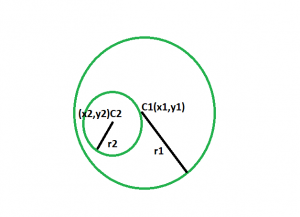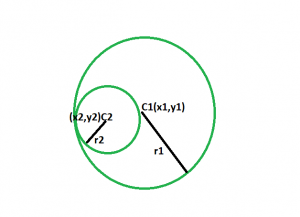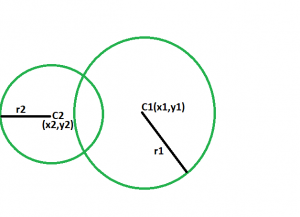# Check if a circle lies inside another circle or not

Given two circles with radii and centres given. The task is to check whether the smaller circle lies inside the bigger circle or not.

Examples:

```Input: x1 = 10, y1 = 8, x2 = 1, y2 = 2, r1 = 30, r2 = 10
Output: The smaller circle lies completely inside
the bigger circle without touching each other
at a point of circumference.

Input :x1 = 7, y1 = 8;x2 = 3, y2 = 5;r1 = 30, r2 = 25
Output :The smaller circle lies completely inside
the bigger circle with touching each other
at a point of circumference.
```

Approach:
Here three cases can come,

•The smaller circle lies completely inside the bigger circle without touching each other at a point of circumference.
If this case happens, the sum of the distance between the centres and smaller radius is lesser than the bigger radius, then obviously the smaller circle lies completely inside the circle, without touching the circumference.
•The smaller circle lies completely inside the bigger circle with touching each other at a point of the circumference. If this case happens, the sum of the distance between the centres and smaller radius is equal to the bigger radius, then obviously the smaller circle lies completely inside the circle, with touching the circumference.
•The smaller does not lies inside the bigger circle completely.If this case happens, then sum of the distance between the centers and smaller radius is greater than the bigger radius, then obviously the smaller circle does not lies completely inside the circle.

Below is the implementation of the above approach:

## CPP

 `// C++ program to check if one circle ` `// lies inside another circle or not. ` ` `  `#include ` `using` `namespace` `std; ` ` `  `void` `circle(``int` `x1, ``int` `y1, ``int` `x2, ` `            ``int` `y2, ``int` `r1, ``int` `r2) ` `{ ` `    ``int` `distSq = ``sqrt``(((x1 - x2) ` `                       ``* (x1 - x2)) ` `                      ``+ ((y1 - y2) ` `                         ``* (y1 - y2))); ` ` `  `    ``if` `(distSq + r2 == r1) ` `        ``cout << ``"The smaller circle lies completely"` `             ``<< ``" inside the bigger circle with "` `             ``<< ``"touching each other "` `             ``<< ``"at a point of circumference. "` `             ``<< endl; ` `    ``else` `if` `(distSq + r2 < r1) ` `        ``cout << ``"The smaller circle lies completely"` `             ``<< ``" inside the bigger circle without"` `             ``<< ``" touching each other "` `             ``<< ``"at a point of circumference. "` `             ``<< endl; ` `    ``else` `        ``cout << ``"The smaller does not lies inside"` `             ``<< ``" the bigger circle completely."` `             ``<< endl; ` `} ` ` `  `// Driver code ` `int` `main() ` `{ ` `    ``int` `x1 = 10, y1 = 8; ` `    ``int` `x2 = 1, y2 = 2; ` `    ``int` `r1 = 30, r2 = 10; ` `    ``circle(x1, y1, x2, y2, r1, r2); ` ` `  `    ``return` `0; ` `} `

## Java

 `// Java program to check if one circle  ` `// lies inside another circle or not.  ` `import` `java.io.*; ` ` `  `class` `GFG  ` `{ ` `         `  `    ``static` `void` `circle(``int` `x1, ``int` `y1, ``int` `x2,  ` `                ``int` `y2, ``int` `r1, ``int` `r2)  ` `    ``{  ` `        ``int` `distSq = (``int``)Math.sqrt(((x1 - x2)  ` `                                    ``* (x1 - x2))  ` `                                    ``+ ((y1 - y2)  ` `                                    ``* (y1 - y2)));  ` `     `  `        ``if` `(distSq + r2 == r1)  ` `        ``{ ` `            ``System.out.println(``"The smaller circle lies completely"` `                ``+ ``" inside the bigger circle with "` `                ``+ ``"touching each other "` `                ``+ ``"at a point of circumference. "``) ; ` `        ``} ` `                 `  `        ``else` `if` `(distSq + r2 < r1)  ` `        ``{ ` `            ``System.out.println(``"The smaller circle lies completely"` `                ``+ ``" inside the bigger circle without"` `                ``+ ``" touching each other "` `                ``+ ``"at a point of circumference."``) ; ` `        ``} ` `                 `  `        ``else` `        ``{ ` `            ``System.out.println(``"The smaller does not lies inside"` `                ``+ ``" the bigger circle completely."``) ; ` `        ``} ` `                 `  `    ``}  ` `     `  `    ``// Driver code  ` `    ``public` `static` `void` `main (String[] args)  ` `    ``{ ` `        ``int` `x1 = ``10``, y1 = ``8``;  ` `        ``int` `x2 = ``1``, y2 = ``2``;  ` `        ``int` `r1 = ``30``, r2 = ``10``;  ` `        ``circle(x1, y1, x2, y2, r1, r2);  ` `    ``} ` `} ` ` `  `// This code is contributed by ajit_00023. `

## Python

 `# Python3 program to check if one circle ` `# lies inside another circle or not. ` ` `  `def` `circle(x1, y1, x2,y2, r1, r2): ` ` `  `    ``distSq ``=` `(((x1 ``-` `x2)``*` `(x1 ``-` `x2))``+` `((y1 ``-` `y2)``*` `(y1 ``-` `y2)))``*``*``(.``5``) ` ` `  `    ``if` `(distSq ``+` `r2 ``=``=` `r1): ` `        ``print``(``"The smaller circle lies completely"` `            ``" inside the bigger circle with "` `            ``"touching each other "` `            ``"at a poof circumference. "``) ` `    ``elif` `(distSq ``+` `r2 < r1): ` `        ``print``(``"The smaller circle lies completely"` `            ``" inside the bigger circle without"` `            ``" touching each other "` `            ``"at a poof circumference. "``) ` `    ``else``: ` `        ``print``(``"The smaller does not lies inside"` `            ``" the bigger circle completely."``) ` ` `  `# Driver code ` `x1 ,y1 ``=` `10``,``8` `x2 ,y2 ``=` `1``, ``2` `r1 ,r2 ``=` `30``,``10` `circle(x1, y1, x2, y2, r1, r2) ` ` `  `# This code is contributed by mohit kumar 29 `

## C#

 `// C# program to check if one circle  ` `// lies inside another circle or not.  ` `using` `System; ` ` `  `class` `GFG ` `{ ` `     `  `    ``static` `void` `circle(``int` `x1, ``int` `y1, ``int` `x2,  ` `                ``int` `y2, ``int` `r1, ``int` `r2)  ` `    ``{  ` `        ``int` `distSq = (``int``)Math.Sqrt(((x1 - x2)  ` `                        ``* (x1 - x2))  ` `                        ``+ ((y1 - y2)  ` `                            ``* (y1 - y2)));  ` `     `  `        ``if` `(distSq + r2 == r1)  ` `        ``{ ` `            ``Console.WriteLine(``"The smaller circle lies completely"` `                ``+ ``" inside the bigger circle with "` `                ``+ ``"touching each other "` `                ``+ ``"at a point of circumference. "``) ; ` `        ``} ` `                 `  `        ``else` `if` `(distSq + r2 < r1)  ` `        ``{ ` `            ``Console.WriteLine(``"The smaller circle lies completely"` `                ``+ ``" inside the bigger circle without"` `                ``+ ``" touching each other "` `                ``+ ``"at a point of circumference."``) ; ` `        ``} ` `                 `  `        ``else` `        ``{ ` `            ``Console.WriteLine(``"The smaller does not lies inside"` `                ``+ ``" the bigger circle completely."``) ; ` `        ``} ` `                 `  `    ``}  ` `     `  `    ``// Driver code  ` `    ``static` `public` `void` `Main () ` `    ``{ ` `        ``int` `x1 = 10, y1 = 8;  ` `        ``int` `x2 = 1, y2 = 2;  ` `        ``int` `r1 = 30, r2 = 10;  ` `        ``circle(x1, y1, x2, y2, r1, r2);  ` `    ``} ` `} ` ` `  `// This code is contributed by AnkitRai01 `

## PHP

 ` `

Output:

The smaller circle lies completely inside the bigger circle without touching each other at a point of circumference.

Attention reader! Don’t stop learning now. Get hold of all the important DSA concepts with the DSA Self Paced Course at a student-friendly price and become industry ready.

My Personal Notes arrow_drop_upBudding Web DeveloperKeen learnerAverage CoderDancer&Social Activist

If you like GeeksforGeeks and would like to contribute, you can also write an article using contribute.geeksforgeeks.org or mail your article to contribute@geeksforgeeks.org. See your article appearing on the GeeksforGeeks main page and help other Geeks.

Please Improve this article if you find anything incorrect by clicking on the "Improve Article" button below.